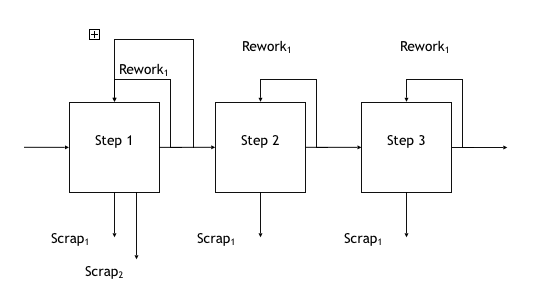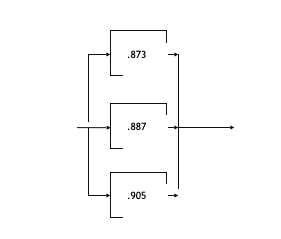First Time Yield and Rolled Throughput Yield are two important standard yield metrics for processes.

First Time Yield (FTY):  The ratio of the number of good “units” coming out of the process to the number of “units” going in.

Rolled Throughput Yield (RTY): The probability that the process produces a good “unit” the first time through.

Unlike First Time Yield, Rolled Throughput Yield explicitly accounts for rework and scrap thus surfacing the “hidden factory.”

In two videos, Academic Gain Tutorials, a free online resource for study materials from many disciplines, describes First Time Yield and Rolled Throughput Yield.

You can view these videos through the listed links for First Time Yield and Rolled Throughput Yield.

First Time Yield

Of course, using the example in the video for the three steps in series, we see that

(95/100)*(85/95)*(80/85) by cancellation = 80/100 simply the definition of First Time Yield, the number of good units exiting divided by the number of units entering.

Rolled Throughput Yield

As described, the RTY calculation is simple if all the process steps are in series and rework is only done once in a given process step.

Suppose however that  rework is done not once but say twice in a process step? And what if some of the process steps are in parallel?

We will address both questions with examples.

First: Rework is done more than onceWe’ll look at the “true” rolled throughput yield focusing on Step 1 for the above example.

We use a subscript to indicate whether scrap or rework is done during the first pass or a second pass, etc.

Step Good Units In Defects Numerator for True Yield

True Yield Out

1 100 10 scrap1  5 rework1 100 – (10 + 5) = 85 85/100 = 0.85

The above is the case that most material simplifies to, only one pass through.

But if we’re really trying to get at the hidden factory and look at the amount of waste, we have to track every pass through a step and take into account whether the results were good or bad.

Let’s assume there was an only second pass through. We could generalize to as many as there are although in practice, we recommend that after a given part is reworked a certain number of times, it should be scrapped.The true throughput yield for Step 1 is therefore 80 percent.

The number of good units going into Step 2 would be 88 = (100 – 12 scrapped).

One would then continue as usual to compute the system Rolled Throughput Yield.

Next: Some process steps are in parallel

Often Lean Six Sigma material only covers RTY for serial operations.

If  we know the individual RTYs for any operation, and we have a combination of series and parallel operations, how do we calculate the RTYoverall for the system?

First, we need some assumptions for the approach we describe.

1. Independence: defects made in one operation do not influence the defects made in another operation
1. In the general case, must all products or services pass through all non-identical parallel operations?

If we know the number of units being processed and the fraction of them going through the different parallel operations, then the RTY for the parallel “block” as a whole is the weighted average of the individual RTYs making up the block.

However, as we will see, the calculations simplify in that if we know the total going in, the total amount of rework, and the total amount of scrap for the block, we can use those numbers to get the RTY for the entire block.

3)  All rework is done by the same operation that produced the defect.

Let us work an example where we know the number of units, how they split among the parallel steps, and the amount of scrap and rework.Legend: RW = Rework, SC = Scrap

We started with 300 units and finished with 275 having scrapped 25 units. However, much rework was done.

The Throughput Yield (YTP) for an operation = (In – RW – SC)/(In)

Therefore YTP1 = (300 – 0 – 12)/300 = 288/300 = 0.96

Similarly,

YTP2  = (288 – 18 – 5)/288 = 265/288 = 0.92

YTP3 = (102 – 10 – 3)/102 = 89/102 = 0.873

YTP4 = (97 – 8 – 3)/97 =  86/97 = 0.887

YTP5 = (84 – 7 – 1)/84 = 76/84 = 0.905

YTP6 = (276 – 27 – 1)/276 = 248/276 = 0.899

We now need to compute the overall RTYSystem

Ops 1, 2, and 6 are in series, and the resultant RTY is computed in the usual way through multiplication.

Ops 3, 4, and 5 are in parallel. As a result, the RTY3, 4, 5 = the weighted average of the individual YTPs weighted by the fraction of units passing through those operations.

RTY3,4, 5 = (102/283)(0.873) + (97/283)(0.887) + (84/283)(0.905) = 0.887

However, if we use the original fractions for the individual YTPs, we see the following:

RTY3,4,5  = (102/283)(89/102) + (97/283)(86/97) + (84/283)(76/284)

But using the obvious cancellation, we have

RTY3,4,5 = 89/283  + 86/283 + 76/283 = 251/283 = 0.887

But this is simply, taking the block as a whole,

RTYBlock  = (INBlock – REWORKBlock – SCRAPBlock)/INBlock

= (283 – 25 – 7)/283 = 251/283 = 0.887

Now we can calculate RTYSystem.

RTYSystem = YTP1*YTP2*RTY3,4,5*YTP6 = 0.96*0.92*0.887*0.899 = 0.704.

What if all we have are the individual YTPs for every operation without the original scrap and rework numbers?  How then do we compute the RTY for a parallel block?If we assumed that the products or services going in were equally split among the operations in the parallel block, which might not be the case, then we could simply average the individual YTPs to get the RTYBlock.

However, since we don’t know that, a better approach is to take the geometric mean of the individual YTPs.

Recall that the geometric mean is simply the nth root of a product of n numbers.

In the above example, then,

RTYBlock = (0.873)( 0.887)(0.905)1/3 = (0.7008)1/3 = 0.888

Of course, in more general cases with series and parallel loops, rework, repair, scrap, etc. a simulation model would probably be required to get a good estimate. This is what Pyzdek recommends – Pzydek, T., The Six Sigma Handbook, McGraw Hill,pp. 488-489, 2003.